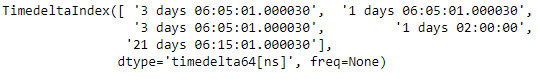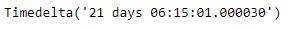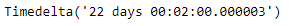# Python | Pandas TimedeltaIndex.min

Python is a great language for doing data analysis, primarily because of the fantastic ecosystem of data-centric python packages. Pandas is one of those packages and makes importing and analyzing data much easier.

Pandas` TimedeltaIndex.min()` function return the minimum value of the TimedeltaIndex object or minimum along an axis.

Syntax : TimedeltaIndex.min(axis=None, *args, **kwargs)

Parameters : None

Return : Timedelta object

Example #1: Use `TimedeltaIndex.min()` function to find the minimum value in the given TimedeltaIndex object.

 `# importing pandas as pd ` `import` `pandas as pd ` ` `  `# Create the TimedeltaIndex object ` `tidx ``=` `pd.TimedeltaIndex(data ``=``[``'3 days 06:05:01.000030'``, ``'1 days 06:05:01.000030'``, ` `                                ``'3 days 06:05:01.000030'``, ``'1 days 02:00:00'``,  ` `                                                 ``'21 days 06:15:01.000030'``]) ` ` `  `# Print the TimedeltaIndex object ` `print``(tidx) `

Output :Now we will use the `TimedeltaIndex.min()` function to find the minimum value in tidx object.

 `# find minimum value ` `tidx.``min``() `

Output :As we can see in the output, the `TimedeltaIndex.min()` function has returned the minimum value in the given TimedeltaIndex object.

Example #2: Use `TimedeltaIndex.min()` function to find the minimum value in the given TimedeltaIndex object.

 `# importing pandas as pd ` `import` `pandas as pd ` ` `  `# Create the TimedeltaIndex object ` `tidx ``=` `pd.TimedeltaIndex(data ``=``[``'06:05:01.000030'``, ``'3 days 06:05:01.000030'``, ` `                                ``'22 day 2 min 3us 10ns'``, ``'+23:59:59.999999'``, ` `                             ``'13 days 06:05:01.000030'``, ``'+12:19:59.999999'``]) ` ` `  `# Print the TimedeltaIndex object ` `print``(tidx) `

Output :Now we will use the `TimedeltaIndex.min()` function to find the minimum value in tidx object.

 `# find minimum value ` `tidx.``min``() `

Output :As we can see in the output, the `TimedeltaIndex.min()` function has returned the minimum value in the given TimedeltaIndex object.

My Personal Notes arrow_drop_upCheck out this Author's contributed articles.

If you like GeeksforGeeks and would like to contribute, you can also write an article using contribute.geeksforgeeks.org or mail your article to contribute@geeksforgeeks.org. See your article appearing on the GeeksforGeeks main page and help other Geeks.

Please Improve this article if you find anything incorrect by clicking on the "Improve Article" button below.

Article Tags :

Be the First to upvote.

Please write to us at contribute@geeksforgeeks.org to report any issue with the above content.